# Triggered Capture Using Preamble Detection

This example shows how to use a software-defined-radio (SDR) to capture data from the air using preamble detection. The example also shows how to use the transmit capabilities of the same radio to loop back a test waveform.

### Introduction

The example demonstrates these steps.

1. Generate a waveform containing a preamble.

2. Configure the preamble detector to detect the preamble sequence.

3. Use the `plotThreshold` function to calibrate a fixed or adaptive threshold and capture data.

4. Explore trigger offset.

### Set Up Radio

Call the `radioConfigurations` function. The function returns all available radio setup configurations that you saved using the Radio Setup wizard. For more information, see Connect and Set Up NI USRP Radios.

`savedRadioConfigurations = radioConfigurations;`

To update the dropdown menu with your saved radio setup configuration names, click Update. Then select the radio to use with this example.

```savedRadioConfigurationNames = [string({savedRadioConfigurations.Name})]; radio =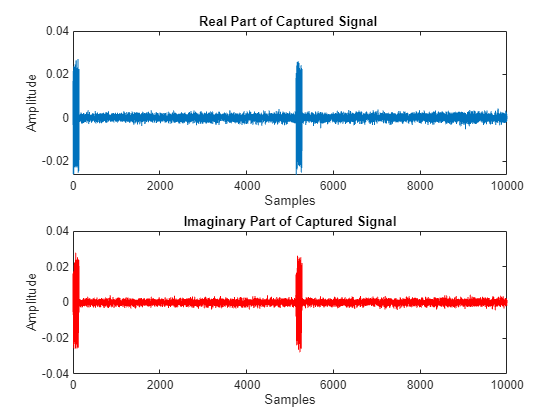savedRadioConfigurationNames(1)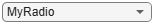;```

### Generate Transmission Waveform

Create a transmission waveform containing a Zadoff-Chu preamble sequence. To enable straightforward demonstration of the preamble detection workflow, concatenate zeros before and after the preamble.

Generate a preamble sequence of length 137 by using 38th root of the Zadoff-Chu sequence and normalize. Concatenate with zeros.

```preamble = zadoffChuSeq(38,137); preamble = preamble/norm(preamble,2); prePadLen = 2501; postPadLen = 2500; headSignal = complex(zeros(prePadLen,1),zeros(prePadLen,1)); rearSignal = complex(zeros(postPadLen,1),zeros(postPadLen,1)); inputSignal = [headSignal; preamble; rearSignal];```

Plot transmission waveform.

```figure(); subplot(2,1,1); plot(real(inputSignal)); subtitle("Real Part"); xlabel("Samples"); ylabel("Amplitude"); title("Waveform with Preamble"); subplot(2,1,2); plot(imag(inputSignal),Color='r'); subtitle("Imaginary Part"); xlabel("Samples"); ylabel("Amplitude");```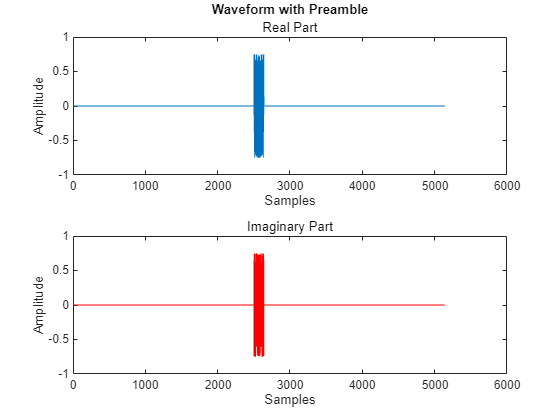### Configure Preamble Detector

Create a preamble detector object with the specified radio. To speed up the execution time of this example upon subsequent runs, reuse the workspace object from the first run of the example.

```clear pd pd = preambleDetector(radio);```

Set the RF properties of the preamble detector. Set the `RadioGain` property according to the local wireless channel.

```pd.SampleRate =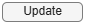30720000; pd.CenterFrequency =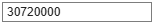2450000000; pd.RadioGain =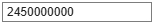30; % Increase if signal levels are low.```

To update the dropdown menu with the antennas available for capture on your radio, call the `hCaptureAntennas` helper function. Then select the antenna to use with this example.

```captureAntennaSelection = hCaptureAntennas(radio); pd.Antennas =captureAntennaSelection(1);```

Configure the preamble sequence for preamble detection.

`pd.Preamble = preamble;`

Set the capture data type to the data type of the generated transmission waveform.

`pd.CaptureDataType = "double";`

### Configure Transmission Variables

Set the transmit gain and transmit antenna values. Set the transmit gain variable according to the local wireless channel.

`txGain =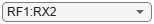30; % Increase if signal levels are low.`

To update the dropdown menu with the antennas available for transmit on your radio, call the `hTransmitAntennas` helper function. Then select the antenna to use with this example.

```transmitAntennaSelection = hTransmitAntennas(radio); txAntenna =transmitAntennaSelection(1); ```

### Detect Preamble Using Fixed Threshold and Capture Data

Data capture is triggered when the correlator output is greater than the fixed threshold. By setting the fixed threshold to 0, you can analyze the behavior of the preamble detector and understand how to set the fixed threshold value for successful detection.

Set the preamble detector to use fixed threshold. To set the threshold method, stop any ongoing transmission.

```stopTransmission(pd); pd.ThresholdMethod = "fixed";```

Set the fixed threshold initially to 0.

`pd.FixedThreshold = 0;`

Transmit the test waveform.

```transmit(pd,inputSignal,"continuous",TransmitGain=txGain, ... TransmitCenterFrequency=pd.CenterFrequency,TransmitAntennas=txAntenna);```

Use the `plotThreshold` function to analyze the behavior of the detector by plotting 10,000 samples. Because the fixed threshold value is 0, all samples from the correlator output are possible trigger points. Check the correlator output values at the peak trigger points. Because the sampling phase determines the quality of the correlator peak, run the `plotThreshold` function multiple times to see how the trigger points change.

`plotThreshold(pd,10e3);`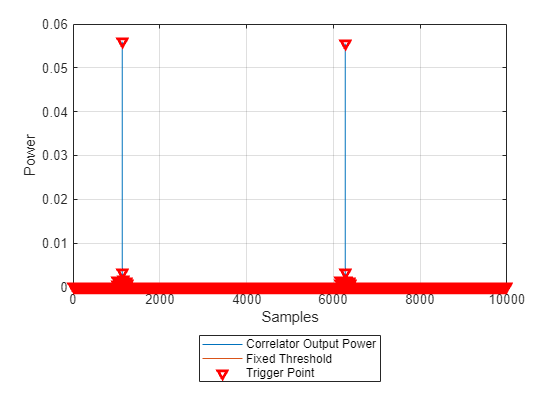Choose a threshold value that is below any of the trigger point values. Plot the threshold information again and adjust the fixed threshold until the trigger points appear only on the correlator output peak.

```pd.FixedThreshold =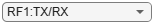5; plotThreshold(pd,10e3);```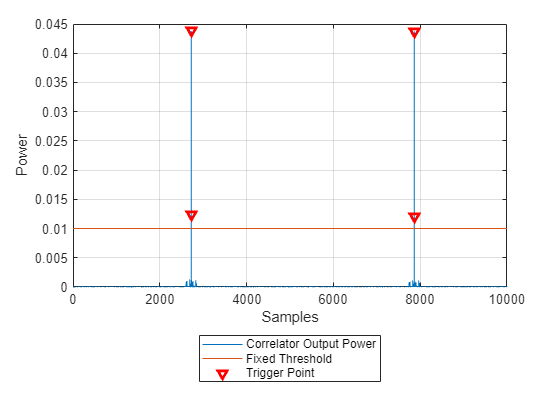Once the threshold is set, capture data.

```[data, ~, ~, status] = capture(pd,10e3,seconds(1)); plotCapturedData(data,status);```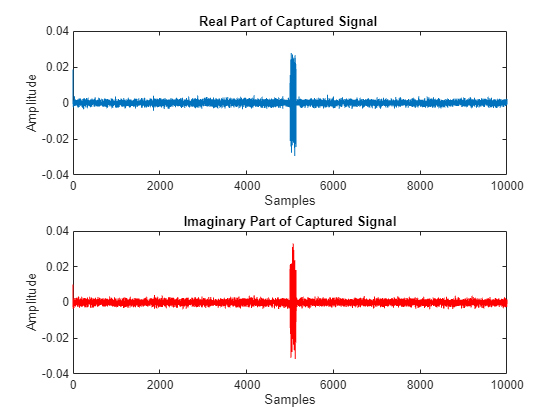### Detect Preamble Using Adaptive Threshold and Capture Data

As an alternative to the fixed threshold, data capture can be triggered when the correlator output is greater than the adaptive threshold, which dynamically varies with the input signal power. By setting the adaptive threshold gain and offset to 0, you can analyze the behavior of the preamble detector and understand how to configure the adaptive threshold for successful detection.

Set the preamble detector to use adaptive threshold. To set the threshold method, stop any ongoing transmission.

```stopTransmission(pd); pd.ThresholdMethod = 'adaptive';```

Set the adaptive threshold gain and offset initially to 0.

```pd.AdaptiveThresholdGain = 0; pd.AdaptiveThresholdOffset = 0; ```

Transmit the test waveform.

```transmit(pd,inputSignal,"continuous",TransmitGain=txGain, ... TransmitCenterFrequency=pd.CenterFrequency,TransmitAntennas=txAntenna);```

Use the `plotThreshold` function to analyze the behavior of the detector by plotting 10,000 samples. Check the correlator output values at the peak trigger points and run the `plotThreshold` function multiple times if necessary.

`plotThreshold(pd,10e3);`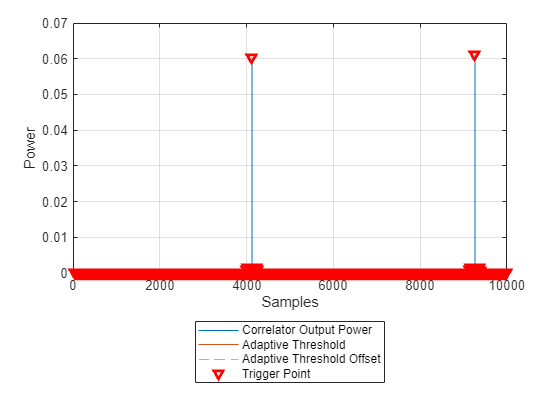To remove all the trigger points from the bottom of the plot, set the adaptive threshold offset to a value that is above the noise floor. Adjust the adaptive threshold gain and plot the threshold information repeatedly until the correlator output is greater than the adaptive threshold.

```pd.AdaptiveThresholdOffset =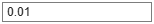1; pd.AdaptiveThresholdGain =0.25; plotThreshold(pd,10e3);```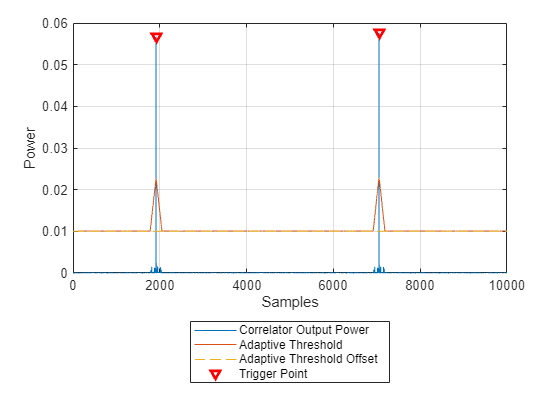Once the threshold is configured, capture data.

```[data, ~, ~, status] = capture(pd,10e3,seconds(1)); plotCapturedData(data,status);```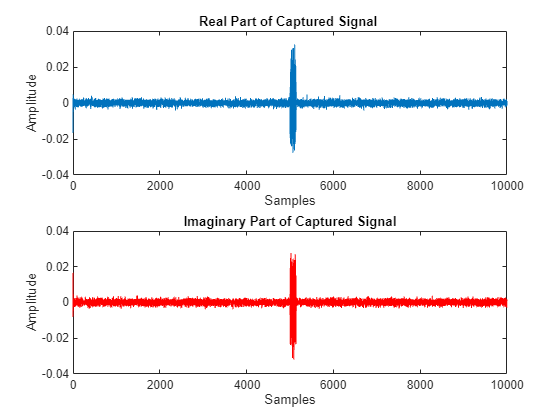### Set Trigger Offset to Include Preamble in Captured Data

To capture the preamble sequence, set the trigger offset to the length of the preamble. To set the trigger offset, stop any ongoing transmission.

```stopTransmission(pd); pd.TriggerOffset =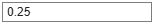-137;```

Transmit the test waveform and capture data.

```transmit(pd, inputSignal,"continuous",TransmitGain=txGain,... TransmitCenterFrequency=pd.CenterFrequency,TransmitAntennas=txAntenna); % Detect and capture 10,000 samples, with a 1 second timeout [data, ~, ~, status] = capture(pd,10e3,seconds(1)); plotCapturedData(data,status);```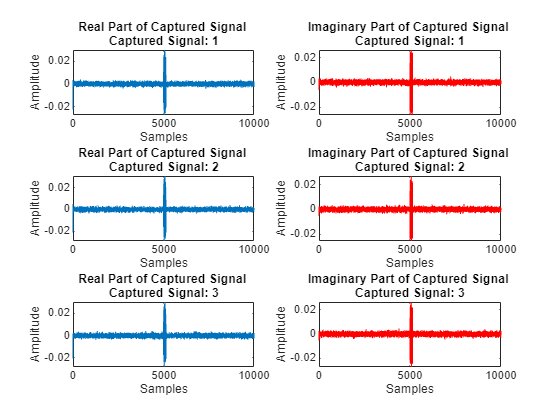### End Transmission

To end the continuous transmission, call the `stopTransmission` function on the preamble detector object.

`stopTransmission(pd);`

### Local Functions

```function plotCapturedData(data,status) if status % If detection is successful, plot data figure(); subplot(2,1,1); plot(real(double(data))); title("Real Part of Captured Signal") xlabel("Samples"); ylabel("Amplitude"); subplot(2,1,2); plot(imag(double(data)),color='r'); title("Imaginary Part of Captured Signal") xlabel("Samples"); ylabel("Amplitude"); else disp("Detection failed.") end end```